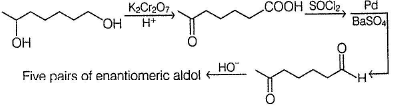Courses

# Test: Aldol Condensation

## 27 Questions MCQ Test Chemistry for JEE | Test: Aldol Condensation

Description
This mock test of Test: Aldol Condensation for JEE helps you for every JEE entrance exam. This contains 27 Multiple Choice Questions for JEE Test: Aldol Condensation (mcq) to study with solutions a complete question bank. The solved questions answers in this Test: Aldol Condensation quiz give you a good mix of easy questions and tough questions. JEE students definitely take this Test: Aldol Condensation exercise for a better result in the exam. You can find other Test: Aldol Condensation extra questions, long questions & short questions for JEE on EduRev as well by searching above.
QUESTION: 1

### Only One Option Correct Type Direction (Q. Nos. 6-15) This section contains 10 multiple choice questions. Each question has four choices (a), (b), (c) and (d), out of which ONLY ONE is correct. Q.  Which is the major product in the following reaction?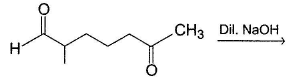Solution: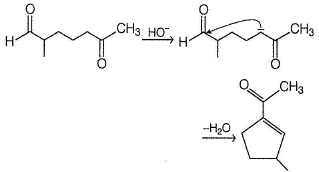QUESTION: 2

### Consider the following reaction,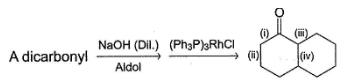Q.  Which of the labeiled C—C bond formation is not possible in the above reaction?

Solution:

An α-βC—C bond is always formed in aidol reaction .

QUESTION: 3

### In a mixed aldoi condensation with ethanal as one aidehyde, which other from the following is expected to give maximum yield of cross condensation product?

Solution:

Nucleophilic addition at carbonyl carbon of cyclopropane releases angle strain.

QUESTION: 4

In the following reaction,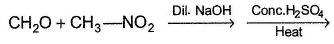The major organic product is

Solution: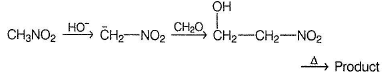QUESTION: 5

Consider the following aldol condensation reaction,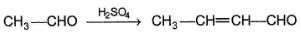Q.

The nucleophile is

Solution:

In acid catalysed aldo! condensation, enol acts as a nucleophile.

QUESTION: 6

In the following reaction,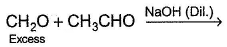Q.

The major final product is

Solution: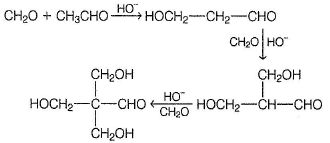Repeated aidol condensation occur due to very high reactivity of formaldehyde towards nucleophile.

QUESTION: 7

Identify the starting reagent needed to make the following compound by mixed aldol condensation.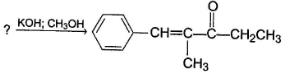Solution: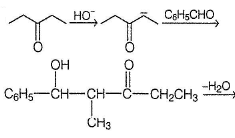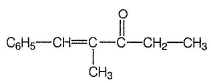QUESTION: 8

The incorrect statement regarding aldol condensation is

Solution:

Enolate is formed in first fast step which undergo nucleophilic addition in second slow step.

QUESTION: 9

Which of the following could result as a product in the aldol condensation reaction?

Solution: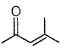It is an α, β-unsaturated ketone which can be formed in an aldol condensation followed by dehydration.

QUESTION: 10

What is the major final product of the following sequence of reaction?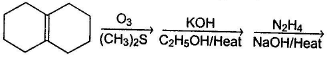Solution: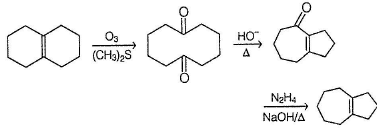*Multiple options can be correct
QUESTION: 11

One or More than One Options Correct Type

Direction (Q. Nos. 11-15) This section contains 5 multiple choice questions. Each question has four choices (a), (b), (c) and (d), out of which ONE or MORE THAN ONE are correct.

Q.

Successful mixed aldol condensation will be favoured

Solution:

If an aldol product is continuously removed from the reaction mixture, reaction occur predominantly in direction of that product (Le-Chatelier’s principle).
Also, if a carbonyl is first treated with strong base like LDA, its role as a nucleophile can be ascertained.

*Multiple options can be correct
QUESTION: 12

Which of the following is/are true regarding aldol condensation ?

Solution:

Both acid and base catalyses aldol reaction. In aldol reaction , a new α - β C—C bond is always formed . α - H is lost in first fast step, hence both CH3CHO and CD3CHO react at the same rate.

*Multiple options can be correct
QUESTION: 13

Which of the following indicated carbon-carbon bond can be formed via intramolecular aldoi condensation of a dicarbonyl compound?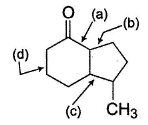Solution:

In aldol reaction as α-β C—C bond is always formed.

*Multiple options can be correct
QUESTION: 14

Which compound (s) below can react via an intramolecular aldol condensation to give a six membered ring?

Solution:

All of these react in aldol reaction giving six-membered ring via intramolecular reaction.
Option (b) gives five and seven membered ring intramolecular aldol condensation.

*Multiple options can be correct
QUESTION: 15

What is(are) true about the following aldoi reaction?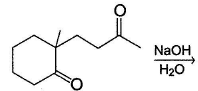Solution: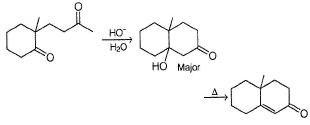Also, the starting compound has three different types of α-H, three different aldols can be formed in principle. If a pure enantiomer of starting compound is taken, a pair of diastereomers of aldol would be formed.

QUESTION: 16

Statement Type

Direction (Q. Nos. 16-19) This section is based on Statement I and Statement II. Select the correct answer from the codes given below.

Q.

Statement I : In aldol condensation, enoiates are nucleophilic and reversibly attack the carbonyl carbon of aldehyde or ketone in the aldoi condensation.

Statement II : An aldoi on refluxing with dilute base gives back the carbonyl compound.

Solution:

Reversibility of aldol into carbonyls establishes that carbanion nucleophile is formed in first fast reversible step.

QUESTION: 17

Statement I : When a mixture of ethanal and propanal is treated with aqueous Na2CO3, four aldol (excluding stereoisomers) are formed.
Statement II : In mixed aldol condensation, two self and two cross condensation products are always formed.

Solution:

It would be true only if both carbonyls are capable of forming enolates, i.e. possesses α-H

QUESTION: 18

Statement I : When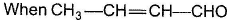is treated with dilute base.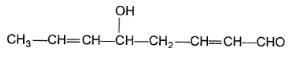is formed.

Statement II : In the given compound, γ-H is most acidic, forms the required enolate.

Solution: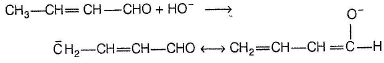QUESTION: 19

Statement I : In the reaction below,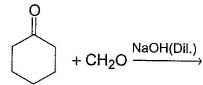A single aldol product is formed in 100% yield.

Statement II : Cross aldol product is formed as major product.

Solution:

Both self-aldol of cyclohexanone (although minor one) and cross aldol are formed.

QUESTION: 20

Comprehension Type

Direction (Q. Nos. 20-22) This section contains a paragraph, describing theory, experiments, data, etc.
Three questions related to the paragraph have been given. Each question has only one correct answer among the four given options (a), (b), (c) and (d).

Passage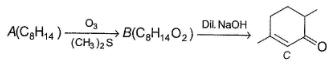A is optically active and C is one of the several aldol possible in the above reaction.

Q.

The structure of A satisfying above criteria is

Solution:

Reversing the final product gives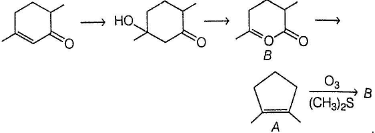QUESTION: 21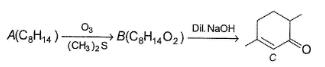A is optically active and C is one of the several aldol possible in the above reaction.

Q.

Besides C, the other six membered cyclic aldol formed in the above reaction is

Solution: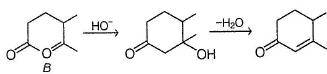QUESTION: 22A is optically active and C is one of the several aldol possible in the above reaction.

Q.

The product B is stereomeric. If a mixture containing all stereoisomers of B is treated with excess of LiAIH4 followed by the acidification will give how many different isomeric diols ?

Solution: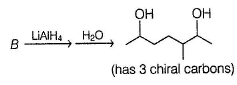*Answer can only contain numeric values
QUESTION: 23

One Integer Value Correct Type

Direction (Q. Nos. 23-27) This section contains 5 questions. When worked out will result in an integer from 0 to 9 (both inclusive).

Consider the following aldol condensation reaction,

Butanone + NaOH (Dil.) → Aldols

Q.

How many different isomers (including stereoisomers) are formed above?

Solution: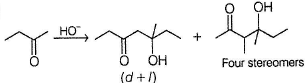*Answer can only contain numeric values
QUESTION: 24

Consider the following cross-aldol condensation reaction,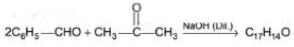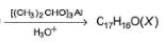Q.

How many different isomeric X are formed?

Solution: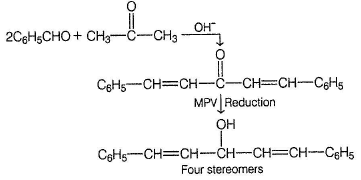*Answer can only contain numeric values
QUESTION: 25

Consider the following modified aldol condensation,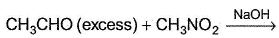Q.

How many ethanal, at the most, will react with one molecule of nitromethane?

Solution:

All three hydrogen can be deprotonated from nitromethane giving

*Answer can only contain numeric values
QUESTION: 26

Consider the following sequence of reaction,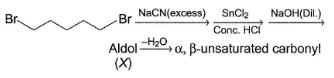Q.

How many different aldol isomers of X are formed?

Solution: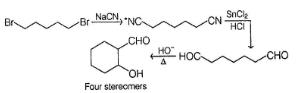*Answer can only contain numeric values
QUESTION: 27

Consider the following sequence of reaction,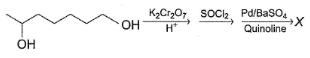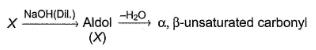Q.

If all undehydrated aldols (X) formed above by intramolecular aldol condensation are considered, how many pairs of enantiomers are formed?

Solution: